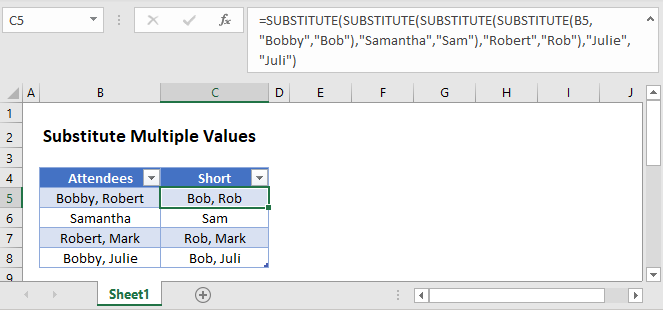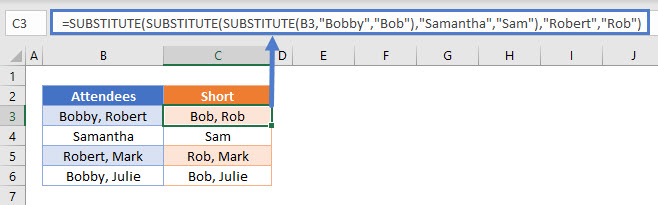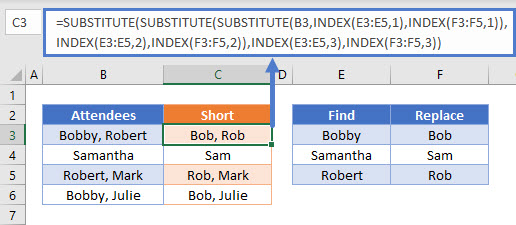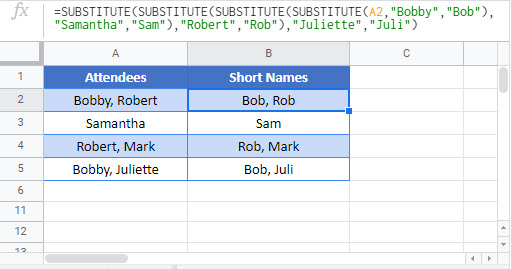# Substitute Multiple Values – Excel & Google Sheets

This tutorial will demonstrate how to find and replace multiple values using nested SUBSTITUTE functions.## Substitute Multiple Values

To find and replace multiple values in Excel, use the SUBSTITUTE function within a nested formula:

``=SUBSTITUTE(SUBSTITUTE(SUBSTITUTE(B3,"Bobby","Bob"),"Samantha","Sam"),"Robert","Rob")``## How does the formula work?

This formula nests multiple SUBSTITUTE functions together to find and replace values one at a time. The result from the first substitution is fed into the next SUBSTITUTE function and so on. In our example, three substitutions are made. To add more substations, simply nest SUBSTITUTE functions in the formula.

## Substitute Multiple Values Using a Reference

Another approach would be to use a reference range instead of typing the values in the formula.

``````=SUBSTITUTE(SUBSTITUTE(SUBSTITUTE(B3,INDEX(\$E\$3:\$E\$5,1),INDEX(\$F\$3:\$F\$5,1)),
INDEX(\$E\$3:\$E\$5,2),INDEX(\$F\$3:\$F\$5,2)),INDEX(\$E\$3:\$E\$5,3),INDEX(\$F\$3:\$F\$5,3))``````This method works the same way as the pervious method except it uses the INDEX function to reference a find and replace range with the values. Two INDEX functions are needed for each SUBSTITUTE (one for the find value and another for the replace value).

## Substitute Multiple Values in Google Sheets

These formulas work exactly the same in Google Sheets as in Excel.### Excel Practice Worksheet

Practice Excel functions and formulas with our 100% free practice worksheets!